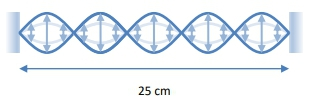## Physics

Learn the toughest concepts covered in Physics with step-by-step video tutorials and practice problems by world-class tutors

18. Waves & Sound

# Standing Waves

1
concept

## Standing Waves8m
Play a video:
2
Problem

In the following figure, what is the harmonic number of the standing wave? The wavelength of the standing wave? If the frequency of the standing wave is 30 Hz, what is the speed of the waves producing the standing wave?3
example

## Unknown Harmonic Frequency4m
Play a video:
Hey, guys, let's do an example. A string fixed at both ends produces a harmonic frequency of hertz, with the next highest harmonic frequency being 780 hertz. If the same, the same string can produce a harmonic frequency of hertz. What harmonic frequency is the next highest. Okay, so we have two different pairs of harmonic frequencies. Initially were saying some harmonic frequency, which I will call F n, which is in V over to El right. It's fixed that both ends. So this is node node, right? So I'm using envy over too well, which is our note note equation. This is 650 hertz. Now I'm saying the next highest, which is in plus one, right? If in was 650 then the next highest is in plus one that's going to be in plus one the over to L, which is 780 hertz. But notice something I Kenbrell up that in plus one into two separate fractions. I can say that in the over to l plus the over to l equals 780 hertz. What's key here? is that this term right here? V over to El. That is how much the frequency grows every time you go up a new harmonic number. So if I'm at 17 and I go up to 18 it's gonna grow by V over to El. So V over 12 is exactly what I need to know to solve the problem. And luckily, I know what envy over to l is right. It's just 650 hertz. So this tells me Hertz plus V over to L, which is our step in the frequency. It's how much the frequency increases by when we increase our harmonic number by one. That's 780 hertz. So V over to l is 7. 80 minus 6 50 which is 130 hertz. Okay, so this is sort of the linchpin to solving that problem. You need to know the step. We don't know anything about the length of the string, the tension, the mass in the string, the speed. We don't know any of that. So we can't actually solve for V over to el. But we found it by comparing one arbitrary harmonic frequency to the next highest. Now we're at some other harmonic frequency. Some other unknown. It really doesn't matter. We'll call it M. And we're saying f of M, which is M V over to l is 390 hertz. Now, the next one above that is in plus one. So this is F M plus one, which is m plus one V over to L. And once again, we can split up this fraction M v over to l plus V over to l. So you see there we've exposed this. Is that increase in frequency whenever you increase the harmonic number by one. This was our original harmonic frequency at M, which was 390 hertz. So this becomes 390 hertz plus that step frequency that we found, plus 130 hertz, which is 520 hurts. And you see, we didn't know anything about this problem. We didn't know how long the string was. We didn't know the speed of the string. We didn't even know what these harmonic numbers were. But all of that is irrelevant because there was enough information to solve for that step that increase in harmonic frequency every time you increase the harmonic number by one. All right, guys, Thanks for watching
4
example

## Standing Wave On A Guitar3m
Play a video: